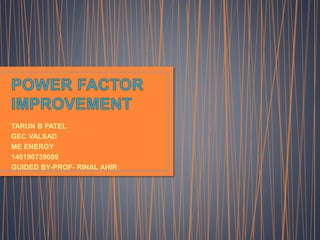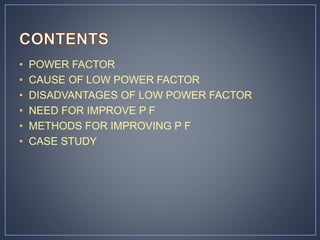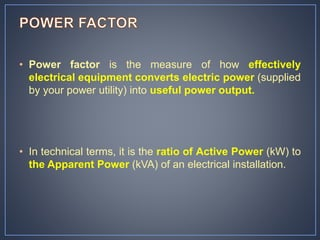Successfully reported this slideshow.×
1 of 25

Power factor improvement

195

Share

ppt contain pf definition,need, disadvantages.methods of improvements,case study

See all

See all

Power factor improvement

1. 1. TARUN B PATEL GEC VALSAD ME ENERGY 140190739009 GUIDED BY-PROF- RINAL AHIR
2. 2. • POWER FACTOR • CAUSE OF LOW POWER FACTOR • DISADVANTAGES OF LOW POWER FACTOR • NEED FOR IMPROVE P F • METHODS FOR IMPROVING P F • CASE STUDY
3. 3. • Power factor is the measure of how effectively electrical equipment converts electric power (supplied by your power utility) into useful power output. • In technical terms, it is the ratio of Active Power (kW) to the Apparent Power (kVA) of an electrical installation.
4. 4. • KW • KVAR • KVA
5. 5. Ø KW (Real Power) KVAR(ReactivePower) = =COS Ø Power Factor (COS Ø) should be close to unity
6. 6. Low power factor results when KW is small in relation to KVA. Remembering our beer mug analogy, this would occur when KVAR (foam, or Mac’s shoulder height) is large. What causes a large KVAR in a system? …….inductive loads 􀂉 Transformers 􀂉 Induction motors 􀂉 Induction generators (wind mill generators) 􀂉 High intensity discharge (HID) lighting
7. 7. Reactive power (KVAR) required by inductive loads increases the amount of apparent power (KVA) in distribution system. This increase in reactive and apparent power results in a larger angle measured between KW and KVA.  As θ increases, cosine θ (or power factor) decreases.
8. 8. KW KVARKVAR ØØ
9. 9. • The PF play important role in a.c circuits since power consumed depends on this factor. • 𝑷 = 𝑽𝑳𝑰𝑳 cos ∅ (FOE SINGLE PHASE) • 𝑰 𝑳 = 𝑷 𝑽 𝑳 𝐜𝐨𝐬 ∅ • 𝑷 = √𝟑𝑽 𝑳 𝑰 𝑳 cos ∅ (FOE THREE PHASE) • 𝑰 𝑳 = 𝑷 √𝟑𝑽 𝑳 𝐜𝐨𝐬 ∅
10. 10. LARGE KVA RATING OF EQUIPMENTS. GREATER CONDUCTOR SIZE. LARGE COPPER LOSSES. POOR VOLTAGE REGULATION. REDUCED HANDLING CAPACITY OF SYSTEM.
11. 11. Lower utility fees by  Reducing peak KW billing demand  Eliminating the power factor penalty Increased system capacity and reduced system losses in your electrical system Increased voltage level in your electrical system and cooler, more efficient motors
12. 12. Sources of Reactive Power (inductive loads) decrease power factor: • Transformers • Induction motors • Induction generators (wind mill generators) • High intensity discharge (HID) lighting Consumers of Reactive Power increase power factor: • Capacitors • Synchronous condenser • Phase advancer
13. 13. Installing capacitors (KVAR Generators) • Installing capacitors decreases the magnitude of reactive power (KVAR or foam), thus increasing your power factor. • Reactive power (KVARS), caused by inductive loads,always acts at a 90-degree angle to working power (KW). • Capacitors store KVARS and release energy opposing the reactive energy caused by the inductor.
14. 14. Minimizing operation of idling or lightly loaded motors. Avoiding operation of equipment above its rated voltage. Replacing standard motors as they burn out with energy-efficient motors
15. 15. A Switchgear Factory supplied with electricity from the distribution utility through 1600 kVA, 11/0.433 kV transformer feeding a Main Low Tension Board (MLTB). The maximum demand of this factory is 1250 kVA at a power factor of 0.75. A 300 KVAR Capacitor Bank was installed at the MLTB bus in this factory to improve the power factor. In this case study, the measurements of Power Factor (PF),Active Power (P), Reactive Power (Q), Apparent Power (S),and Current (I) were illustrated during 12 working hours (from 06:00 to 18:00 hrs) in a day time before and after operating the Capacitor Bank that was installed at the MLTB.
16. 16. • The average power factor improved by 21% as it was 0.75 before PFC and became 0.95 after PFC. • The average loading on the transformer released by 26% as it was 372 kVA before PFC and became 296 kVA after PFC. • The losses of the cable reduced by 36% as the average current passing through the cable was 497 A before PFC and became 395 A after PFC. • The capacitor compensated 61% of the consumed reactive power as the average was 245 kVAR before PFC and became 96 kVAR after PFC.
17. 17. • Osama A. Al-Naseem and Ahmad Kh. Adi,” Impact of Power Factor Correction on the Electrical Distribution Network of Kuwait – A Case Study”, The Online Journal on Power and Energy Engineering (O,JPEE). Vol. (2) – No. (1) • Sapna Khanchi, Vijay Kumar Garg,” Power Factor Improvement of Induction Motor by Using Capacitors”, International Journal of Engineering Trends and Technology (IJETT) – Volume 4 Issue 7- July 2013. • Powerfactor basic.pdf
18. 18. •THANK YOU.

Editor's Notes

• During recent years, increasing attention has been paid to minimize the energy cost and inefficiency in electricity generation, transmission and distribution
In recent years, energy auditing and the power quality of the AC system has become a great concern due to the rapidly increased energy demand and numbers of electronic equipment, power electronics and high voltage power system, In order to reduce the energy consumption and harmonic contamination in power lines and improve transmission efficiency, power factor correction research became a hot topic
• Power factor improvement may be defined as the ratio of the real power to the apparent power. It is a dimensionless quantity whose value lies between 0 and 1.
• KW is Working Power (also called Actual Power or Active Power or Real Power).It is the power that actually powers the equipment and performs useful work.
KVAR is Reactive Power.
It is the power that magnetic equipment (transformer, motor and relay) needs to produce the magnetizing flux.
KVA is Apparent Power.
It is the “vectorial summation” of KVAR and KW.
Let’s look at a simple analogy in order to better understand these terms….
Let’s say you are at the hotel and it is a really hot day. You order up a mug of your favorite beer. The thirst-quenching portion of your beer
is represented by KW (Figure 1).
Unfortunately, life isn’t perfect. Along with your ale comes a little bit of foam. (And let’s face it…that foam just doesn’t quench your thirst.) This
foam is represented by KVAR.
The total contents of your mug, KVA, is this summation of KW (the beer) and KVAR (the foam).
So, now that we understand some basic terms, we are ready to learn about power factor:
Power Factor (P.F.) is the ratio of Working Power to Apparent Power.
Looking at our beer mug analogy above, power factor would be the ratio of beer (KW) to beer plus foam (KVA).
Thus, for a given KVA:
• The more foam you have (the higher the percentage of KVAR), the lower your ratio of KW (beer) to KVA (beer plus foam). Thus, the lower your power factor.
• The less foam you have (the lower the percentage of KVAR), the higher your ratio of KW (beer) to KVA (beer plus foam). In fact, as your foam (or KVAR) approaches zero, your power factor approaches 1.0.
• Let’s look at another analogy ……
Mac here is dragging a heavy load (Figure 2). Mac’s Working Power (or Actual Power) in the forward direction, where he most wants his load to
travel, is KW.
Unfortunately, Mac can’t drag his load on a perfect horizontal (he would get a tremendous backache), so his shoulder height adds a little Reactive
Power, or KVAR.
The Apparent Power Mac is dragging, KVA, is this “vectorial summation” of KVAR and KW.
The “Power Triangle” (Figure 3) illustrates this relationship between KW, KVA, KVAR, and Power Factor:
In order to have an “efficient” system (whether it is the beer mug or Mac dragging a heavy load), we want power factor to be as close to 1.0 as possible.
Sometimes, however, our electrical distribution has a power factor much less than 1.0. Next, we’ll see what causes this.
• These inductive loads constitute a major portion of the power consumed in industrial complexes.
• Reactive power (KVAR) required by inductive loads increases the amount of apparent power (KVA) in your distribution system (Figure 4). This increase in reactive and apparent power results in a larger angle θ (measured between KW and KVA).
Recall that, as θ increases, cosine θ (or power fac tor) decreases
So, inductive loads (with large KVAR) result in low power factor.
• In a c circuit there is generally a phase difference @ between voltage and currnent. the tern cosfive is called pf of the circuit.\
If the circuit is inductives,the current lags behind the voltages and pf is referred lagging.
In capacitance circuit,current lead the voltages and pf is to be leading.
Its aususlly a practise to attach the word lagging and leading with the numerical value of pf .if circiut has pf 0.8 means there is a 0.8lagging .
Some times its expressed in percentages.
• DISADVANTAGES OF LOW POWER FACTOR
Its clear from the above that for fixed power and voltage, the load is inversly proportional to the power factor.lower the power factor,higher is the load and vice –versa.a pf less than unity results in following disadvantages
• The electrical machinery(altenator,tranformer,switch gear) is always rated in kva.
Kva =kw/ cos ∅
It is clear that kva rating of the equipment is inversly proprtional to pf.
The smaller the pf.the larger is the kva rating.there for at low pf, the kva rating of the equipment has to be made more.making the equipment larger and expensive.

***the ele m/c is rated kva the pf of not known when its manufactured in the company.
To transmmit or distribute fixed amount of power at constant voltage,the conductor will carry more current at low power factor.this neccesited large coductor size.
****
large curent at low pf causes more losses.
****the larger curent at lagging pf cause a greater voltage drop in trasformer,alternator,transmission line and disrtibuton.thus imparing on perforemance of equipements.additional voltage regulator is required.
****bcoz reactive component of current prevents the full utilization of capacity of system.

• You want to improve your power factor for several different reasons. Some of the benefits of improving your power factor include:
By raising your power factor, you use less KVAR. This results in less KW, which equates to a rupees savings from the utility.
Utilities usually charge customers an additional fee when their power factor is lessThus, you can avoid